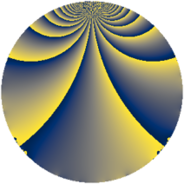# Properties

 Label 847.2.iLevel $847$ Weight $2$ Character orbit 847.i Rep. character $\chi_{847}(241,\cdot)$ Character field $\Q(\zeta_{6})$ Dimension $128$ Newform subspaces $3$ Sturm bound $176$ Trace bound $1$

# Related objects

## Defining parameters

 Level: $$N$$ $$=$$ $$847 = 7 \cdot 11^{2}$$ Weight: $$k$$ $$=$$ $$2$$ Character orbit: $$[\chi]$$ $$=$$ 847.i (of order $$6$$ and degree $$2$$) Character conductor: $$\operatorname{cond}(\chi)$$ $$=$$ $$77$$ Character field: $$\Q(\zeta_{6})$$ Newform subspaces: $$3$$ Sturm bound: $$176$$ Trace bound: $$1$$ Distinguishing $$T_p$$: $$2$$

## Dimensions

The following table gives the dimensions of various subspaces of $$M_{2}(847, [\chi])$$.

Total New Old
Modular forms 200 160 40
Cusp forms 152 128 24
Eisenstein series 48 32 16

## Trace form

 $$128q + 6q^{3} + 56q^{4} + 54q^{9} + O(q^{10})$$ $$128q + 6q^{3} + 56q^{4} + 54q^{9} - 12q^{12} - 16q^{14} + 20q^{15} - 56q^{16} + 10q^{23} + 16q^{25} - 78q^{26} - 6q^{31} + 32q^{36} - 12q^{37} + 78q^{38} - 64q^{42} - 84q^{45} + 24q^{47} - 10q^{49} - 44q^{56} + 22q^{58} - 12q^{59} - 52q^{60} - 20q^{64} + 8q^{67} + 38q^{70} - 4q^{71} + 102q^{75} - 76q^{78} - 54q^{80} + 4q^{81} - 78q^{82} + 50q^{86} + 42q^{89} + 50q^{91} - 40q^{92} + 16q^{93} + O(q^{100})$$

## Decomposition of $$S_{2}^{\mathrm{new}}(847, [\chi])$$ into newform subspaces

Label Dim. $$A$$ Field CM Traces $q$-expansion
$$a_2$$ $$a_3$$ $$a_5$$ $$a_7$$
847.2.i.a $$24$$ $$6.763$$ None $$0$$ $$0$$ $$0$$ $$0$$
847.2.i.b $$48$$ $$6.763$$ None $$0$$ $$6$$ $$0$$ $$0$$
847.2.i.c $$56$$ $$6.763$$ None $$0$$ $$0$$ $$0$$ $$0$$

## Decomposition of $$S_{2}^{\mathrm{old}}(847, [\chi])$$ into lower level spaces

$$S_{2}^{\mathrm{old}}(847, [\chi]) \cong$$ $$S_{2}^{\mathrm{new}}(77, [\chi])$$$$^{\oplus 2}$$